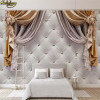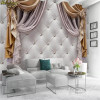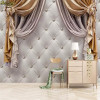# beibehang Custom European curtain soft bag Photo Wallpaper Living Room Bedroom Sofa Background Painting Background 3D wall paper

(No reviews yet)
Condition:
New
Availability:
Imported
Options available
Shipping:
Calculated at Checkout
••••••MSRP: ₹2,499
₹1,268
— You save ₹1,231

## Description

• Function:Mould-Proof
• Function:Waterproof
• Function:Soundproof
• Function:Sound-Absorbing
• Function:Anti-static
• Function:Moisture-Proof
• Function:Smoke-Proof
• Function:Heat Insulation
• Material:Silk wallpaper
• Surface Treatment:Printed
• Usage:Household
• Usage:Commerce
• Usage:Entertainment
• Feature:Environment Friendly
• Feature:Extra Thick
• Feature:Mildew Resistant
• Feature:Formaldehyde-free
• Feature:Water Resistant
• Brand Name:beibehang
• Use:Elders' Room
• Use:Living Room
• Use:Kitchen
• Use:Wedding House
• Use:Study
• Use:Bedding Room
• Model Number:16276972
• Type:Natural Material Wallpapers
• Natural Material Wallpaper Material:Cork / Bark
• is_customized:Yes
• Style:Modern
• Pattern:Geometric Pattern
• Charge Unit:Yuan/Roll

mural wallpaper price: \$ / square meter.    Not rolls.

1 square meter = 1pcs.   2 square meter=2pcs.   3 square meter =3pcs ...

Width x height = square meters = Order Quantity
Note: Please be careful to order 1m2. Because a very small size

We have 16 fixed size order.

Quantity: 1  = 1 s  quare meter      =  140cm (W) x 70cm (H) (4'3 "x 2'3") ft
Quantity: 2  = 2 s  quare meter     =   200cm (W) x 100cm (H) (6'5 "x 3'2") ft
Quantity: 3  = 3     s  quare meter     =   208cm (W) x 146cm (H) (6'8 "x 4'8") ft
Quantity: 4  = 4     s  quare meter       =    200cm (W) x 200cm (H) (6'5 '' x 6'5 '') ft
Quantity: 5  = 5      s  quare meter        =   250cm (W) x 200cm (H) (7'6 '' x6'5 '') ft
Quantity: 6 = 6      s  quare meter         =   300cm (W) x 200cm (H) (9'8 '' x 6'5 '') ft
Quantity: 7 =7     s  quare meter       =    300cm (W) x 250cm (H) (9'8 "x 8'2") ft
Quantity: 8 =8      s  quare meter       =    400cm (W) x 200cm (H) (13'6 "x 6'5") ft
Quantity: 9  =9       s  quare meter       =    350cm (W) x 260cm (H) (11'4 "x 8'7") ft
Quantity: 10 = 10      s  quare meter      =    360cm (W) x 280cm (H) (11'8 "x 9'2") ft
Quantity: 11  = 11    square meter   =  420cm(W) x 260cm(H) (13'9'' x 8'6'') ft

Quantity: 12   =   12     square meter  =   400cm (W) x 300cm (H) (13'6 "x 9'8") ft
Quantity: 13   =   13     square meter  =    460cm(W) x 280cm(H) (15'1'' x 9'2'') ft

Quantity: 14   =   14      square meter  =    480cm(W) x 290cm(H) (15'9'' x 9'6'') ft

Quantity: 15   =  15      square meter   =    500cm(W) x 300cm(H) (16'5'' x 9'10'') ft
Quantity: 16 = 16      s quare meter     =    500cm (W) x 320cm (H) (16'4 "x 10'5") ft

Other size . (width and height) . please tell us.

We will give you calculate the correct order quantity and price

FAQ: buyers ordered to leave. No size information. what to do?
We will wait for buyers 24 hours.
We will send          corresponding          default 16 fixed size.

FAQ:How to order other sizes?
Order other Size steps:
1: Select the correct quantity = square meter (width x height)